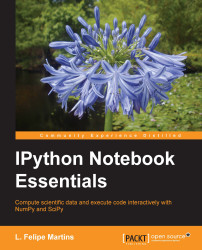•#### IPython Notebook Essentials#### Overview of this book

IPython Notebook EssentialsCreditswww.PacktPub.comPrefaceFree Chapter
A Tour of the IPython NotebookThe Notebook InterfaceGraphics with matplotlibHandling Data with pandasAdvanced Computing with SciPy, Numba, and NumbaProIPython Notebook Reference CardA Brief Review of PythonNumPy ArraysIndex## Advanced mathematical algorithms with SciPy

In this section, we will cover some of the algorithms available in `SciPy`. Each of the following subsections features a representative example from a significant area of applied science. The examples are chosen so as not to require extensive domain knowledge but still be realistic. These are the topics and examples that we present:

• Solving equations and finding optimal values: We will study a market model that requires the solution of a nonlinear system and a facility location problem requiring a nonstandard optimization.

• Calculus and differential equations: We will present a volume calculation that uses integral calculus, and Newton's canon, a thought experiment proposed by Isaac Newton, which we will model using a system of differential equations. Finally, we will present a three-dimensional system, the famous Lorenz equations, which is an early example displaying chaotic behavior.

### Solving equations and finding optimal values

To illustrate this...